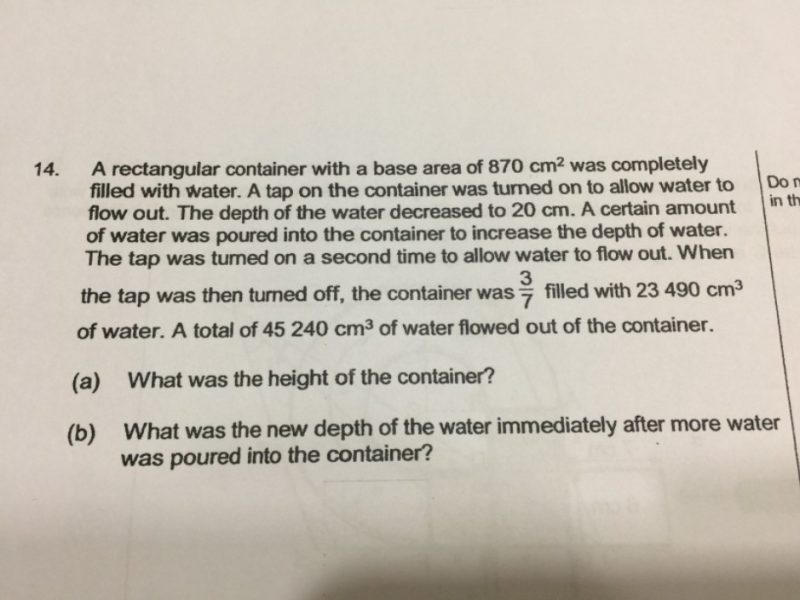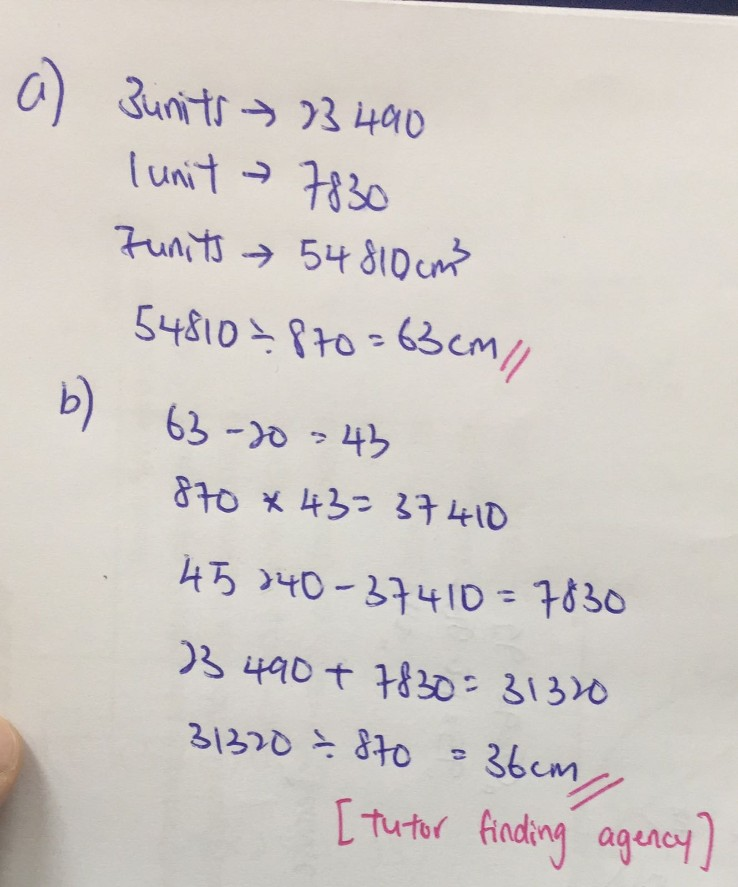# QuestionInitially, the container was full.

Final position, the container was 3/7 filled with 23490 cm³.

7/7 —> 23490/3 × 7 = 54810

54810/870 = 63

(a) The height of the container was 63 cm.

The depth of the water decreased to 20 cm.

63 cm – 20 cm = 43 cm

Volume of water that flowed out = 43 × 870 = 37410 cm³

Total volume of water that flowed out = 45240 cm³

Additional volume of water that flowed out = 45240 – 37410 = 7830 cm³

7830/870 = 9

The final depth of water was 3/7 × 63 cm = 27 cm.

(b) The new depth of water after more water was poured in was 27 cm + 9 cm = 36 cm.

To recap, this was what happened

At first, the depth of water was 63 cm.

Then it dropped by 43 cm to 20 cm after the tap was turned on.

Then more water was poured in till the depth increased by 16 cm to 36 cm.

The tap was turned on till the depth decreased by 9 cm to 27 cm.